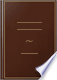Αναζήτηση Εικόνες Χάρτες Play YouTube Ειδήσεις Gmail Drive Περισσότερα »
Είσοδος
 Βιβλία Βιβλία 1 - 10 από 154 για Any two angles of a triangle are together less than two right angles..Any two angles of a triangle are together less than two right angles.Geometry Without Axioms; Or the First Book of Euclid's Elements. With ... - Σελίδα 70
των Thomas Perronet Thompson - 1833 - 150 σελίδες
Πλήρης προβολή - Σχετικά με αυτό το βιβλίο## Elements of Geometry: Containing the First Six Books of Euclid, with a ...

John Playfair - 1806 - 311 σελίδες
...-the angle ACD, is greater than the angle ABC. Therefore, if one side, &c. Q. E, D. PROP. XVII. THEOR. ANY two angles of a triangle are together less than two right angles. Let ABC be any triangle j any two of its angles together are less than two right angles. Produce BC to D; and...## The Elements of Euclid: Viz. the First Six Books, Together with the Eleventh ...

Euclid, Robert Simson - 1806 - 518 σελίδες
...d, the angle ACD, is greater than the angle ABC. Therefore, if one side, &c. QED PROP. XVII. THEOR. ANY two angles of a triangle are together less than two right angles. A Let ABC be any triangle ; any two of its angles together are less than two right angles. Produce...## Elements of Geometry, Geometrical Analysis, and Plane Trigonometry: With an ...

Sir John Leslie - 1809 - 493 σελίδες
...angle BCF (Def. 10.), and hence BCF must be greater than either the angle CBA-orCAB, PROP. XI. THEOR. Any two angles of a triangle are together less than two right angles. The two angles BAC and BCA of the triangle ABC are together less than two right angles. For produce...## The Elements of Euclid: Viz. the First Six Books, Together with the Eleventh ...

Euclid - 1810 - 518 σελίδες
...d, the angle ACD, is greater than the angle ABC. ThereTore, if one side, &c. QED PROP. XVII. THEOR. ANY two angles of a triangle are together less than two right angles. Let ABC be any triangle; any A . two of its angles together are less than two right angles. . Produce BC to D;...## The Elements of Euclid: Viz. the First Six Books, Together with the Eleventh ...

Euclides - 1816 - 528 σελίδες
...is*, the angle ACD, is greater than the angle ABC. Therefore, if one side,&c. QED PROP. XVII. THEOR. ANY two angles of a triangle are together less than two right angles. Let ABC beany triangle;any two of its angles together are less than two right angles. Produce BC to D ; and...## Elements of Geometry and Plane Trigonometry: With an Appendix, and Copious ...

Sir John Leslie - 1817 - 432 σελίδες
...triangle are greater than the interior, and likewise greater than three right angles. PROP. IX. THEOR. Any two angles of a triangle are together less than two right angles. The two angles BAC and BCA of the triangle ABC are, together less than two right angles. For produce...## Elements of Geometry: Containing the First Six Books of Euclid: With a ...

John Playfair - 1819 - 317 σελίδες
...the angle ACD, is greater than the angle ABC. Therefore, if one side, &c. Q, ED PROP. XVII. THEOR. - Any two angles of a triangle are together less than' two right angle*. Let A BC be any triangle ; any two of its angles together are less than two right angles. Produce...## The Youth's instructer [sic] and guardian

1854
...proved hereafter, it is here inserted in order to facilitate the theory of parallel lines. Cor. 2. Any two angles of a triangle are together less than two right angles. For either is less than the supplement of the other. Cor. 3. If any angle of a triangle is right or...## A Popular Course of Pure and Mixed Mathematics ...: With Tables of ...

Peter Nicholson - 1825 - 372 σελίδες
...1.) the angle ACD, is greater than the angle ABC. Therefore, if one side, &c. Q. Ë. D. Proposiiio» XVII. Theorem. Any two angles of a triangle are together less than two right angles. Let ABC be any triangle ; any two of its angles together are less than two right angles. Produce BC to D ; and...## Elements of arithmetic, algebra and geometry

George Lees - 1826 - 207 σελίδες
...that the angle BCG, that is, ACD% is e 5. 1. greater than ABC. Therefore, if one side, &c. QED Cor. Any two angles of a triangle, are together less than two right angles. For, since by the proposition, the exterior angle ACD is greater than the interior and opposite angle...